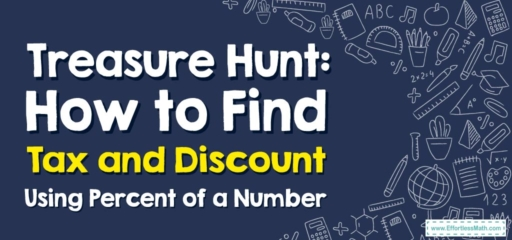# Treasure Hunt: How to Find Tax and Discount Using Percent of a Number

On today's adventure, we're not looking for hidden treasures but hidden savings! Tax and discount are two important factors in determining the final price of a product. Grab your compass and map; it's time to delve into the exciting world of percentages!## 1. X Marks the Spot: Understanding Tax and Discount

Before we set sail, let’s understand these two key terms:

• Tax: An additional amount of money, usually a percentage, added to the purchase price.
• Discount: A reduction in price, often expressed as a percentage off the original price.

## 2. Sailing for Savings: How to Find Tax and Discount

With our treasure map in hand, let’s navigate the seas of savings!

### Treasure Guide: Finding Tax and Discount

#### Step 1: Identify the Original Price

First, identify the original price of the item. This will be the listed price before tax and discount.

#### Step 2: Calculate the Discount

If a discount is offered, calculate the amount saved by multiplying the original price by the discount rate.

#### Step 3: Calculate the Tax

Then, calculate the tax by multiplying the price after the discount (if there is one) by the tax rate.

For instance, if you find a treasure map priced at $$50$$ with a $$20$$$$\%$$ discount and a $$5$$$$\%$$ sales tax, how much would you pay?

1. Identify the Original Price: The map costs $$50$$.
2. Calculate the Discount: The discount is $$20$$$$\%$$ of $$50$$, or $$10$$. So the price after the discount is $$50\ – 10 = 40$$.
3. Calculate the Tax: The tax is $$5$$$$\%$$ of $$40$$, or $$2$$. So the final price is $$40 + 2 = 42$$.

There you have it, treasure hunters! You’ve successfully navigated the complex seas of taxes and discounts. Always remember, the path to the best deals might not be a straight line, but with a bit of calculation and deduction, you’ll find your way! Happy hunting!

### What people say about "Treasure Hunt: How to Find Tax and Discount Using Percent of a Number - Effortless Math: We Help Students Learn to LOVE Mathematics"?

No one replied yet.

X
30% OFF

Limited time only!

Save Over 30%

SAVE $5 It was$16.99 now it is \$11.99